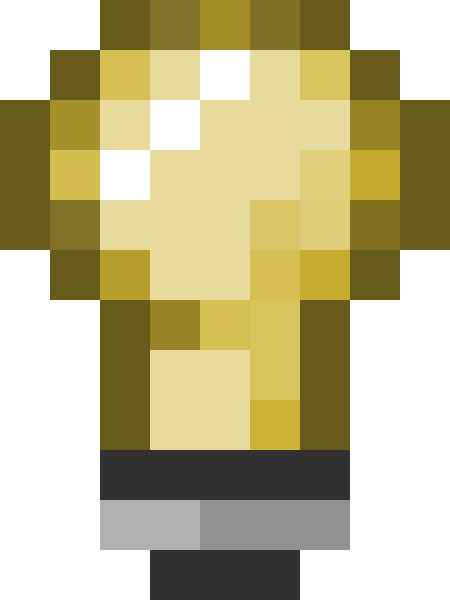# Difference between revisions of "Matrix3x4 t"

## Contents

`matrix3x4_t` is a C++ class that represents a matrix: a mathematical construct that allows Vectors to be transformed.

Matrices are chiefly used to rotate vectors, since translation and scaling are taken care of by vector addition and multiplication respectively. A single matrix can store a transformation in all three modes however, making them useful in transformation-intense operations like vertex rendering and 3D view set-up.

To do: What is `VMatrix`, and what relationship does it have to this class?

## Functions

### Generation

Matrix generation is best left to Source's pre-existing functions:

```void AngleMatrix( QAngle angle, matrix_3x4_t& out ); // Rotation; can also use Quaternions or Radians
void PositionMatrix( Vector position, matrix3x4_t& out ); // Translation
void AngleMatrix( QAngle angle, Vector position, matrix3x4_t& out ); // Rotation + translation
void SetScaleMatrix( float scale, matrix3x4_t& out ); // Scale
```

Use `MatrixMultiply()` to combine two matrices into one. You can do this any number of times.

### ApplicationTip: Unless re-using an existing matrix you are better off rotating a vector with `VectorRotate()`, which accepts angles directly.

The mathematical notation for a matrix transformation is (vector * matrix). Source does not support that syntax, though it would be trivial to add if you so wanted, and instead offers these functions:

```void VectorTransform( Vector in1, matrix3x4_t in2, Vector& out );
void VectorITransform( Vector in1, matrix3x4_t in2, Vector& out ); // 'Inverse' of the above
```

There is an overload of `VectorRotate()` that accepts a `matrix3x4_t`. It seems to behave in the same way as `VectorTransform()`.

### Other / utility

`void MatrixAngles()`
`void MatrixPosition()`
`void MatrixVectors()`
Extract angles and vectors from a matrix. `MatrixAngles()` has many overloads. There is no way to extract a scale.
`void MatrixTranspose()`
Transposes a matrix. To do: What this is good for.
`void MatrixInvert()`
Reverses a matrix, making it transform in the opposite direction(s). You could also use `VectorITransform()` to apply the matrix.
`bool MatricesAreEqual()`
`void MatrixCopy()`Confirm:Instead of (matrix1 = matrix2).
• `Vector`
• `QAngle`
• `Quaternion`
• `RadianEuler`